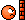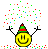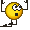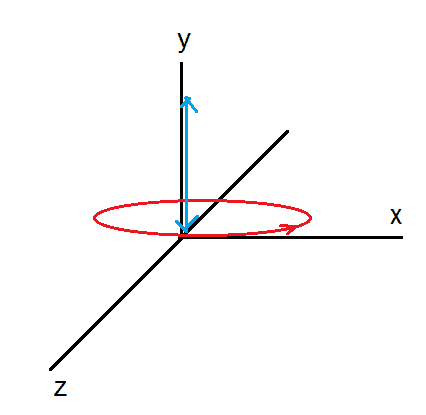# Charged particle in Electric and Magnetic field

ehild
Homework Helper
Sorry . I think It should be ##x=\frac{v_1m}{qB}sinωt## only . I don't see why there should be a minus sign .
x is correct. I meant z, sorry.

•Vibhor
##z = - \frac{v_1m}{qB}(1-cosωt) ## ??

ehild
Homework Helper
##z = - \frac{v_1m}{qB}(1-cosωt) ## ??
Yes.

•Vibhor
Ok .

x will be 0 when ##\frac{qBt}{\pi m }## is an integer , y will be 0 at ##t = \frac{2mv_2}{qE}## , z will be 0 when ##\frac{qBt}{2\pi m }## is an integer

ehild
Homework Helper
Ok .

x will be 0 when ##\frac{qBt}{\pi m }## is an integer , y will be 0 at ##t = \frac{2mv_2}{qE}## , z will be 0 when ##\frac{qBt}{2\pi m }## is an integer
What integer is it? When is z = 0? And all of them should be zero at the same time.

•Vibhor
Option B)ehild
Homework Helper
Option B)Correct. Was it difficult?

•Vibhor
Was it difficult?

It surely was until you took over. Honestly speaking , I had almost made up my mind to leave this problem as I was completely clueless. But last couple of hours of problem solving were pretty exciting,thanks to you .

You are amazing.

ehild
Homework Helper
It was the combination of a circular motion in the x,z plane and a vertical projectile motion in the direction of y. The period of the circular motion should be the same as the flight time of the vertical projectile.Thank you for the thanksLast edited:
•Vibhor